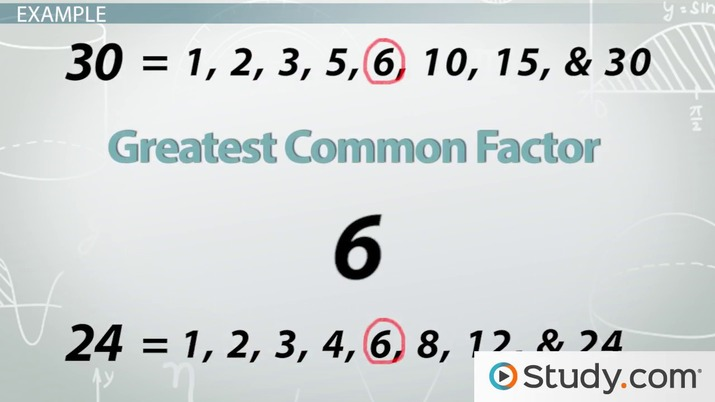# Word Problems: Greatest Common Factor & Least Common Multiple

Lesson Transcript
Instructor: Yuanxin (Amy) Yang Alcocer

Amy has a master's degree in secondary education and has taught math at a public charter high school.

In this video lesson, you will learn what kinds of word problems you can expect to see that involve the greatest common factor or the least common multiple. Learn what you need to do to understand these problems and then to solve them.

## Greatest Common Factor

Word problems, here we go! Yes, in this video lesson, we will be learning about word problems. Now, don't get all worried. I know that word problems are sometimes the hardest part of math. But I'll show you how you can solve these word problems without breaking your brain.

The first type of word problem that we will consider in this video lesson is the one that involves the greatest common factor, the greatest common factor that is shared between two or more numbers. For example, the number 3 is the greatest common factor between 9 and 6 because both can be divided equally by 3. There is no higher number that can be divided equally between 9 and 6.An error occurred trying to load this video.

Try refreshing the page, or contact customer support.

Coming up next: What is Factoring in Algebra? - Definition & Example

### You're on a roll. Keep up the good work!

Replay
Your next lesson will play in 10 seconds
• 0:01 Greatest Common Factor
• 0:46 Example - GCF
• 2:23 Least Common Multiple
• 2:57 Example - LCM
• 3:54 Lesson Summary
Save Save

Want to watch this again later?

Timeline
Autoplay
Autoplay
Speed Speed

## Example

When you come across a word problem that involves the greatest common factor, you might see a problem like this one:

'Farmer John and Farmer Jane are planning out their fruit orchard. Farmer John is planting the orange trees, and Farmer Jane is planting the cherry trees. Farmer John has 30 orange trees to plant, and Farmer Jane has 24 cherry trees to plant. They want to plant the trees so that each row has the same number of trees. What is the largest number of trees each row can have?'

This problem is a greatest common factor word problem because it is asking you to find the greatest common factor between the numbers 30 and 24. The greatest common factor in our word problem translates to the number of trees in each row. Do you remember how to find the greatest common factor? The easiest method is to write out all the factors for each number. Then, we compare the factors and find the greatest factor that they both have in common.

So, the number 30 has the factors 1, 2, 3, 5, 6, 10, 15, and 30. The number 24 has the factors 1, 2, 3, 4, 6, 8, 12, and 24. Now, which of these numbers do they have in common? They have 1, 2, 3, and 6 in common. Which one is the greatest? 6 is the greatest, so 6 is the greatest common factor; that means 6 is our answer. We can have a maximum of 6 trees in each row of the fruit orchard so that all the rows are the same number.

To unlock this lesson you must be a Study.com Member.

### Register to view this lesson

Are you a student or a teacher?